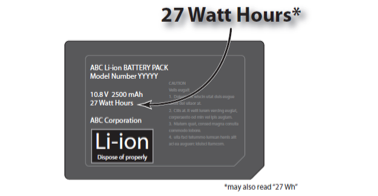# How to calculate Battery Watt-Hour (WH) RatingIf you're a frequent traveller, you may be aware of that whether or not a lithium battery can be brought on board depends on its Watt-Hour (WH) rating. But what exactly is WH, and where do you get this information?

The best place to look would be on the battery itself. Sometimes, the WH rating is stated quite clearly, like in the picture above.

But much of the time, it is not stated. The way to calculate this is to use the voltage of the battery, and multiply it by the capacity.

Voltage is denominated in Volts (V), while capacity is denoted in Ampere-Hours (AH). In the above example, the battery is 10.8V, while the capacity is 2,500 mAH. Since 1 AH is equal to 1,000 mAH (milli-Ampere-Hours), the capacity is 2.5AH.

Therefore, the WH rating on this battery is 10.8 V x 2.5 AH = 27 WH.

---------------------------------------------------------------

Buy the world's lightest motorized mobility chair!